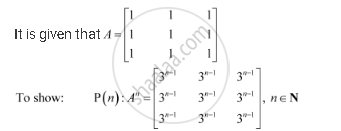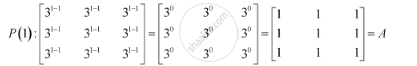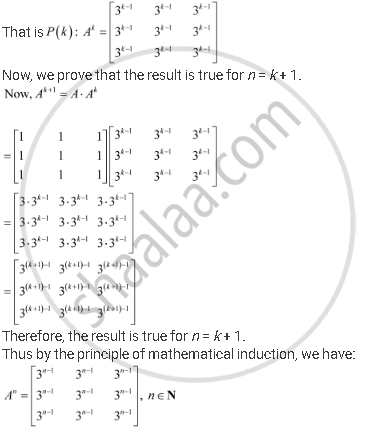Share

# If a = Matric [(1,1,1),(1,1,1),(1,1,1)], Prove that A" N∈N - CBSE (Science) Class 12 - Mathematics

#### Question

if A = [(1,1,1),(1,1,1),(1,1,1)], Prove that A" = [(3^(n-1),3^(n-1),3^(n-1)),(3^(n-1),3^(n-1),3^(n-1)),(3^(n-1),3^(n-1),3^(n-1))] n in N

#### SolutionWe shall prove the result by using the principle of mathematical induction.

For n = 1, we have:Therefore, the result is true for n = 1.

Let the result be true for n = k.Is there an error in this question or solution?

#### Video TutorialsVIEW ALL 

Solution If a = Matric [(1,1,1),(1,1,1),(1,1,1)], Prove that A" N∈N Concept: Types of Matrices.
S